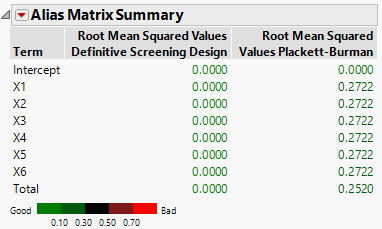Publication date: 05/24/2021

## Alias Matrix Summary

The alias matrix addresses the issue of how terms that are not included in the model affect the estimation of the model terms, if they are indeed active. In the Alias Terms outline, you list potentially active effects that are not in your assumed model but that might bias the estimates of model terms. The alias matrix entries represent the degree of bias imparted to model parameters by the Alias Terms effects. See Alias Terms and Alias Matrix.

The Alias Matrix Summary table lists the terms in the assumed model. These are the terms that correspond to effects listed in the Model outline. Given a design, for each entry in the Term column, the square root of the sum of the squared alias matrix entries for the terms corresponding to effects in the Alias Terms outline is computed. This value is reported in the Root Mean Squared Values column for the given design. For an example, see Example of Calculation of Alias Matrix Summary Values.

Note: The Alias Matrix Summary report appears only if there are effects in the Alias Terms list.

Figure 16.27 Alias Matrix Summary for Two DesignsFigure 16.27 shows the Alias Matrix Summary report for the Plackett-Burman and Definitive Screening designs constructed in Designs of Same Run Size, with only main effects in the Model outline. All two-factor interactions are in the Alias Terms list. The table shows that, for the Definitive Screening Design, main effects are uncorrelated with two-factor interactions.

The Root Mean Squares Values are colored according to a color gradient shown under the Alias Matrix Summary table. You can control the color legend using the options in the Alias Matrix Summary red triangle menu. See Color Dashboard.

### Alias Matrix

The rows of the Alias Matrix are the terms corresponding to the model effects listed in the Model outline. The columns are terms corresponding to effects listed in the Alias Terms outline. The entry in a given row and column indicates the degree to which the alias term affects the parameter estimate corresponding to the model term.

In evaluating your design, you ideally want one of two situations to occur relative to any entry in the Alias Matrix. Either the entry is small or, if it is not small, the effect of the alias term is small so that the bias is small. If you suspect that the alias term might have a substantial effect, then that term should be included in the model or you should consider an alias-optimal design. In fact, alias-optimality is driven by the squared values of the alias matrix.

### Example of Calculation of Alias Matrix Summary Values

This example illustrates the calculation of the values that appear in the Alias Matrix Summary outline. In this example, you compare the two designs assuming that only main effects are active.

1. Select Help > Sample Data, click Open the Sample Scripts Directory, and select Compare Same Run Size.jsl.

2. Right-click in the script window and select Run Script.

Two 13-run design tables are constructed:

Definitive Screening Design

Plackett-Burman

You are interested only in the Plackett-Burman design. This is the active table.

3. From the Plackett-Burman table, select DOE > Design Diagnostics > Evaluate Design.

4. Select X1 through X6 and click X, Factor.

5. Click OK.

6. Open the Alias Terms outline to confirm that all two-factor interactions are in the Alias Terms list.

7. Open the Alias Matrix outline.

For each model term listed in the Effect column, the entry in that row for a given column indicates the degree to which the alias term affects the parameter estimate corresponding to the model term.

For example, to obtain the Alias Matrix Summary entry in Figure 16.27 corresponding to X1, square the terms in the row for X1 in the Alias Matrix, average these, and take the square root. You obtain 0.2722.